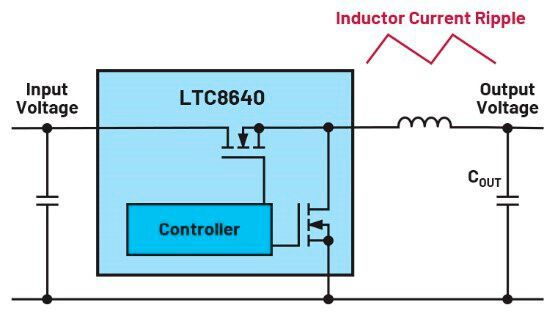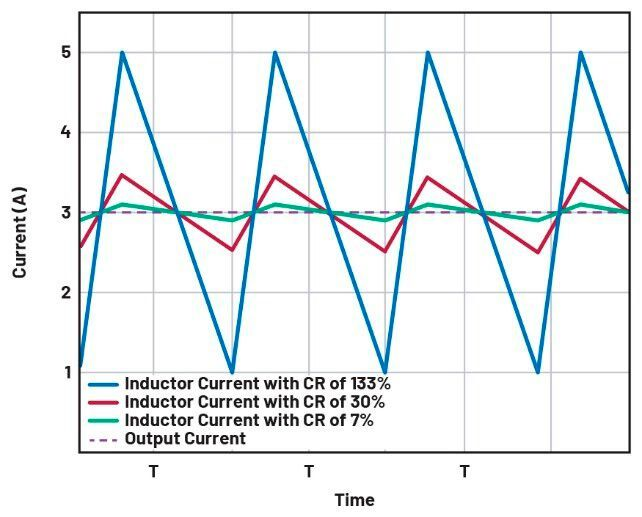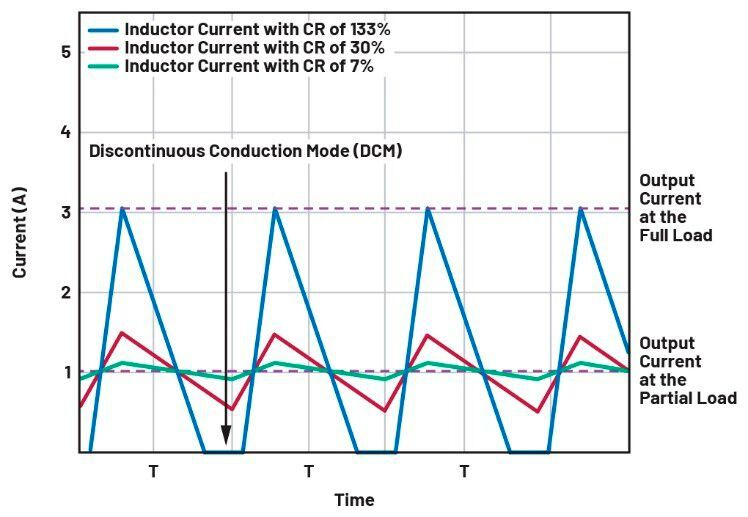#### Power Management Expert Frederik Dostal

Subject Matter Expert - Power Management, Analog Devices GmbH

## INDUCTOR CURRENT RIPPLESelecting the right inductor current ripple

From Frederik Dostal* Reading Time: 4 min

Related Vendors

A switching regulator converts an input voltage to a higher or lower output voltage. For this, an inductor is used to store energy temporarily. The size of this inductor depends on the switching frequency of the switching regulator as well as the expected current flow through the circuit.

How exactly is the correct inductance value selected? It can be done using a commonly used formula that contains the inductor current ripple. In the data sheets for most switching regulators and in most application notes and other explanatory texts, an inductor current ripple of 30 % during nominal load operation is given as a recommendation. This means that at the nominal load current, the inductor current peaks lie 15 % above and the inductor current valleys lie 15 % below the average current. Why is this inductor current ripple, or current ripple ratio (CR), of 30 % usually selected as a good compromise?Image 1. A buck converter with an inductor current ripple. (Source: Frederik Dostal)

For a buck converter such as the one shown in Image 1, Equation 1 applies:

This equation calculates the required inductor value L for a buck converter based on the current ripple ratio CR. This ratio is usually specified as 0.3, or 30 %, peak-to-peak ripple. Here, D is the duty cycle and T is the cycle time, which depends on the respective switching frequency.

### What happens with different inductor current ripples?Image 2. An inductor current ripple with a ripple current ratio of 30 % shown in red, with a smaller inductor in blue, and with a large inductor in green at the nominal load. (Source: Frederik Dostal)

An inductor current ripple with a ripple current ratio of 30 % shown in red, with a smaller inductor in blue, and with a large inductor in green at the nominal load.

Figure 2 shows the inductor current ripple (current ripple ratio (CR)) of 30 % in a circuit with an output current of 3 A in red. This is the compromise that is usually selected in switching regulator circuit designs. The waveform shown in blue has an inductor current ripple of 133 %, and the waveform shown in green has an inductor current ripple of 7 %.Image 3. An inductor current ripple with a ripple current ratio of 30 % shown in red, with a small inductor in blue, and with a large inductor in green at the partial load. (Source: Frederik Dostal)

Image 3 shows what happens when the same circuit is operated with part of the nominal load as the output current such as 1 A. At a high inductor current ripple, shown by the blue line in Figure 3, the energy in the inductor is completely discharged in every cycle. This mode is called discontinuous conduction mode (DCM). In this mode, the control loop stability behavior changes and a higher output voltage ripple can occur.

A certain ripple current ratio is needed in order for DCM to be avoided. With a ripple current ratio of 30 %, a good compromise is achieved. If the ripple current ratio is low, even at partial loads, the system is mainly operating in continuous current conduction mode. The circuit can thus be optimized for operation in this mode.

### What if the selected ripple current ratio is too high?

At high ripple current ratios of greater than 30 %, the inductor is smaller and thus cheaper. Unfortunately, the peak currents increase dramatically and generate more electromagnetic interference (EMI) than is absolutely necessary in a typical circuit. In addition, continuous conduction mode (CCM) can only be reached at higher load currents. This does not have to be a problem, but the operating behavior in this mode is changed and must be taken into account in the circuit design. Higher output voltage ripple than for a lower inductor current ripple also result.

### What if the effects of selecting a ripple current ratio that is too low?

For low ripple current ratios of less than 30 %, the inductor is large and accordingly expensive. The load transient response is also slower due to the large size of the energy storage device. If, for example, a high load current is disconnected rapidly, the energy stored in the inductor has to go somewhere. This increases the voltage across the output capacitor (COUT). The more energy in the inductor, the higher the output voltage excess. This excess voltage can damage the supplied circuit.

If the advantages and disadvantages of different inductor current ripple ratios are weighed, then values of around 30 % seem to represent a good trade-off for most applications. However, deviations are permissible in some cases as long as the resulting effects are acceptable.

Have you enjoyed reading this article? Then follow us on LinkedIn and stay up-to-date with daily posts about the latest developments on the industry, products and applications, tools and software as well as research and development.